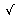# Binary Color Device

On two separate occasions (Magic Squares and Nim) I had an opportunity to discuss the Binary System and Boolean Algebra. These two algebraic structures that both use only two digits (0 and 1) to represent their elements, are still much different. Any number can be represented in the Binary System. Only two values are dealt with in the Boolean Algebra. These two values, true and false are often denoted by 1 and 0, respectively. Another essential difference is that quite different sets of operations are defined for the two structures.

As with any number system, addition, subtraction, multiplication, division, square root are defined in the Binary System. They are carried out much the same as in the Decimal or any other number system. 1 + 1 = 10, 1 × 1 = 1, 10 × 10 = 100,100 = 10, etc. In the Boolean Algebra, one normally defines conjunction (&, AND), disjunction (V, OR), negation (~, NOT), exclusive OR (^, XOR), and more. Just for the completeness sake, I'll repeat the definitions here.

 & 0 1 0 0 0 1 0 1
 V 0 1 0 0 1 1 1 1
 ^ 0 1 0 0 1 1 1 0

So far I hope the difference is clear. Now let's make a further step. Given a set of numbers, say, all real numbers R, we may consider a set of pairs R2 = {(x,y): x,y both real}. We may also consider triples (R3 = {(x,y,z): x,y,z real}) and n-tuples (Rn={(x1,...,xn): x1,...,xn real}). This would be the beginning of Analytical and Multidimensional Geometry. Defining a set of componentwise operations on the tuples, we convert the sets Rn into vector spaces. For example,

(x1, y1) + (x2, y2) = (x1 + x2, y1 + y2).

This approach of constructing new algebraic structures from the old ones is known as the Direct Sum method. It's quite general and, in particular, applies to Boolean Algebras. In other words, if B = {0, 1} is a Boolean algebra, we may consider a set of n-tuples Bn = {(x1, ..., xn): x1, ..., xn boolean}. Logical operations are defined componentwise, as usual. For example (0, 1)^(1, 1) = (0^1, 1^1) = (1,0), etc.

In the case of Boolean direct sums, it's possible to employ a more cryptic notation writing a tuple one component at a time without parentheses and commas. For example, (1, 1, 0, 1) can be written as 1101; and (0, 1, 1, 0) as 110 if it's clear what's size tuples we are dealing with.

Now for the last step. The string 1101 may be looked at as a binary representation of the (decimal) number 13 for (1101)2 = 1310. Also 1102 = 610 which means that 110 is the binary representation of the decimal number 6. Thus decimal (or actually numbers in any other system) numbers may be (and often are) looked at as representations of multidimensional Boolean Algebras. Every component of a tuple, when the latter is written as just described (without parentheses or commas), are commonly referred to as bits.

Operations of conjunction, disjunction, etc. are said to apply bitwise to regular integers. For example,

 1310 & 610 = 11012 & 1102 = (1, 1, 0, 1) & (0, 1, 1, 0) = (1 & 0, 1 & 1, 0 & 1, 1 & 0) = (0, 1, 0, 0) = 1002 = 410.

Bitwise operations are especially useful for storing and manipulating colors in computers. In paint programs or in programs that allow some kind of multiple object selection, you often draw what's known as a rubber band rectangle, by dragging the mouse. The shimmering rectangle which appears to follow the cursor, is actually drawn by a double application of XOR. This is based on the following nice property a^b^b = a.

Below is a simple puzzle that may help you to get familiar with the bitwise operations. We consider B4, a four-dimensional boolean space. In all, 16 tuples can be represented using four binary digits (bits). Thus we use numbers 0 through 15 to represent these tuples. The purpose of the game is to get all 16 squares filled with the right numbers which are written left to right and top to bottom beginning with 0 in the upper left box. The game starts by pressing the New Puzzle button. You may drag any square that has been assigned color and drop it on any other square. If the latter has also been assigned color, the bitwise operation that shows in the combobox will apply to the new assignment.

### If you are reading this, your browser is not set to run Java applets. Try IE11 or Safari and declare the site https://www.cut-the-knot.org as trusted in the Java setup.What if applet does not run?